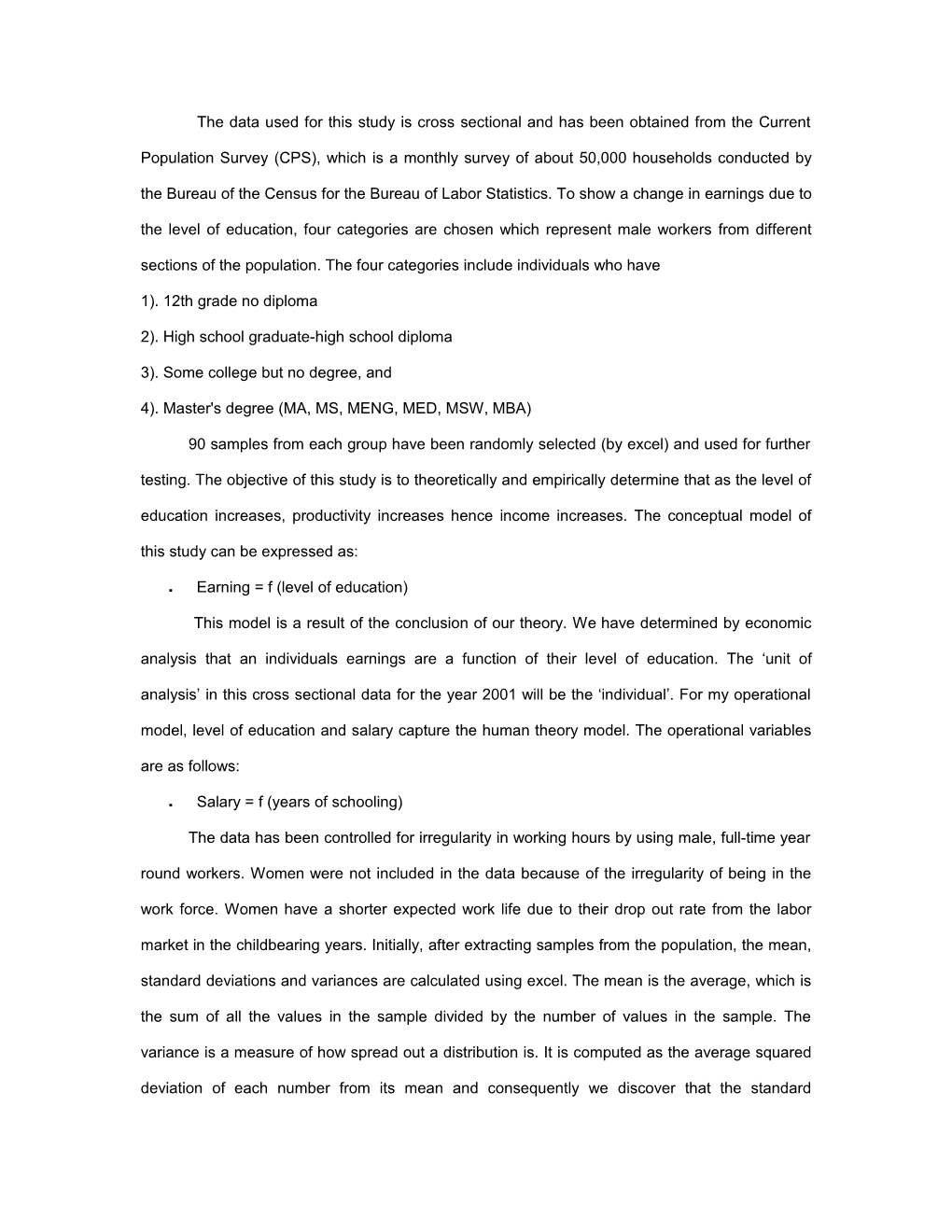# The Data Used for This Study Is Cross Sectional and Has Been Obtained from the CurrentThe data used for this study is cross sectional and has been obtained from the Current Population Survey (CPS), which is a monthly survey of about 50,000 households conducted by the Bureau of the Census for the Bureau of Labor Statistics. To show a change in earnings due to the level of education, four categories are chosen which represent male workers from different sections of the population. The four categories include individuals who have

2). High school graduate-high school diploma

3). Some college but no degree, and

4). Master's degree (MA, MS, MENG, MED, MSW, MBA)

90 samples from each group have been randomly selected (by excel) and used for further testing. The objective of this study is to theoretically and empirically determine that as the level of education increases, productivity increases hence income increases. The conceptual model of this study can be expressed as:

• Earning = f (level of education)

This model is a result of the conclusion of our theory. We have determined by economic analysis that an individuals earnings are a function of their level of education. The ‘unit of analysis’ in this cross sectional data for the year 2001 will be the ‘individual’. For my operational model, level of education and salary capture the human theory model. The operational variables are as follows:

• Salary = f (years of schooling)

The data has been controlled for irregularity in working hours by using male, full-time year round workers. Women were not included in the data because of the irregularity of being in the work force. Women have a shorter expected work life due to their drop out rate from the labor market in the childbearing years. Initially, after extracting samples from the population, the mean, standard deviations and variances are calculated using excel. The mean is the average, which is the sum of all the values in the sample divided by the number of values in the sample. The variance is a measure of how spread out a distribution is. It is computed as the average squared deviation of each number from its mean and consequently we discover that the standard deviation is the positive square root of the variance.The logic behind hypothesis testing is that if the result obtained from the samples is significantly different from that of the hypothesized population value, then the hypothesis should be rejected. In order to test if the two populations have equal variances we do an F-test. In my particular analysis all the three categories as described in the ‘hypothesis’ section have been dealt with individually. The idea of calculating the categories separately is to determine if the variances for both the sections selected are from the same population and whether it is reasonable to assume that the two population means are equal. For all the categories in my example we choose the level of significance to be 5%, i.e. 95% level of confidence. The F test statistic can be calculated by the formula:

• F = s21

s2 2

Where s2 = sample standard deviation.

To determine whether our theory holds true with our given data we need to check if our critical-F is less than or greater than the F-statistic. To calculate the F statistic we use the ‘FINV’ formula in excel (results in Table 2 of Appendix). The ‘FINV’ formula finds the critical F using the probability (in our case 0.05) and the combination of both the degrees of freedom. For every individual test that we calculated, it was noted that the F-stat was less than the Critical F. In all the three categories, at a 95% confidence level our Critical F values of 1.419 are greater than the F-stat values of ‘1’. With the results of the F-test statistic we conclude that our population variances are equal. In order to now prove our theory we move on to calculating the critical value of the t-statistic.